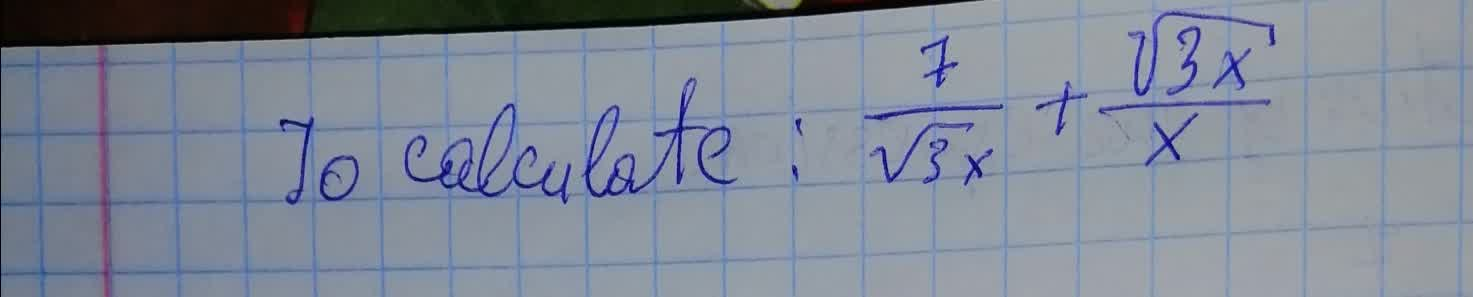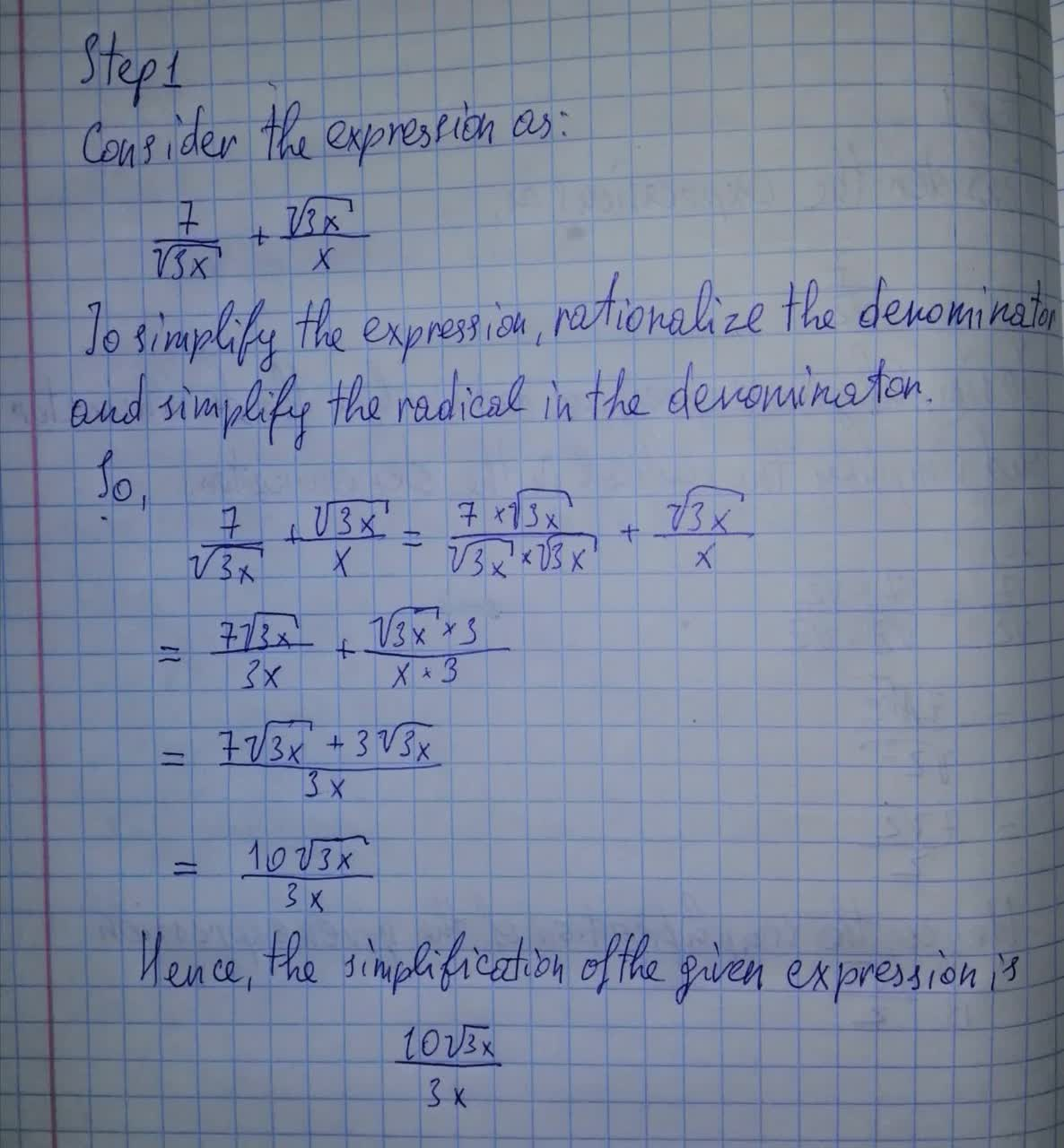Question# To calculate: The simplification of the expression \frac{7}{\sqrt{3x}}+\frac{\sqrt{3x}}{x}

Conic sections
ANSWEREDTo calculate: The simplification of the expression $$\displaystyle{\frac{{{7}}}{{\sqrt{{{3}{x}}}}}}+{\frac{{\sqrt{{{3}{x}}}}}{{{x}}}}$$